|

# 吊顶,究竟该吊不该吊?

我觉得如果建筑物本身的梁、管道看得见的话可以做吊顶遮丑。我家走廊做了局部吊顶，就是因为有3根梁，遮丑，做完之后的确是空间比较流畅。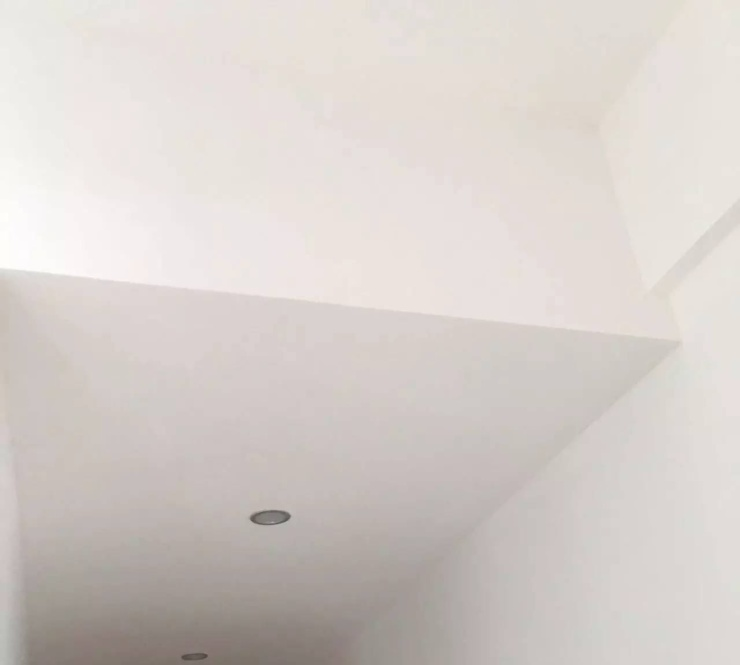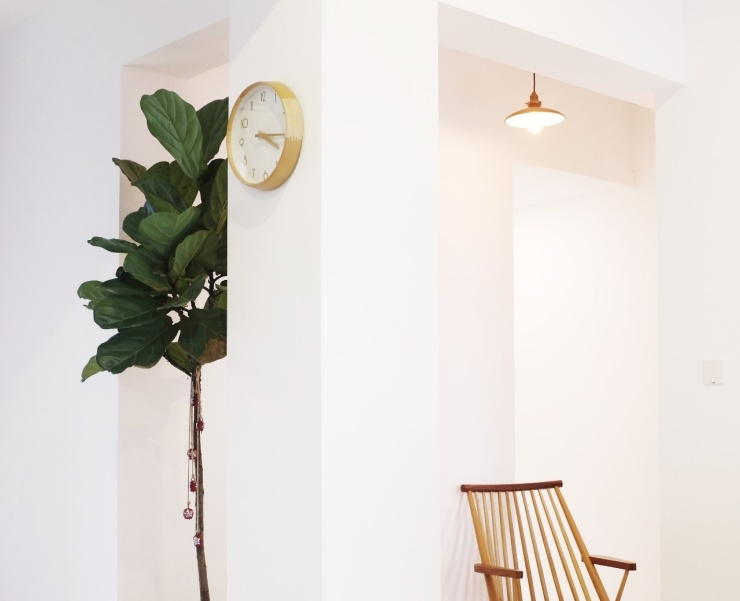好好住APP用户路路通

（还在装修中）卫生间、厨房需要吊顶。另外，我家新风和中央空调的管道需要，所以迫不得已才做了吊顶，也只是做的局部吊顶。后期为了不太突兀，整个空间全部用白色硅藻泥，灯具也选择了白色+亚克力结合的超薄灯具，白色吊灯，感觉自己非常用心的在弱化上方的存在感。‍‍

‍‍

好好住APP用户东卿

我还觉得如果在老房子里，隔音效果不是很好，可以考虑做吊顶，中间填充隔音材料。

好好住APP用户刘一汀

要不要吊顶也要根据一个空间想表达出的空间语言、空间要求和功能性要求。比如就说要藏管线和梁，很多人说做圈顶，做圈顶的原因就是要藏起来空调和新风的管线。如果没有功能性要求 不建议做吊顶，国内住宅层高在2.4m~2.7m，豪宅顶配层高也就3.3m，不吊顶空间更舒适。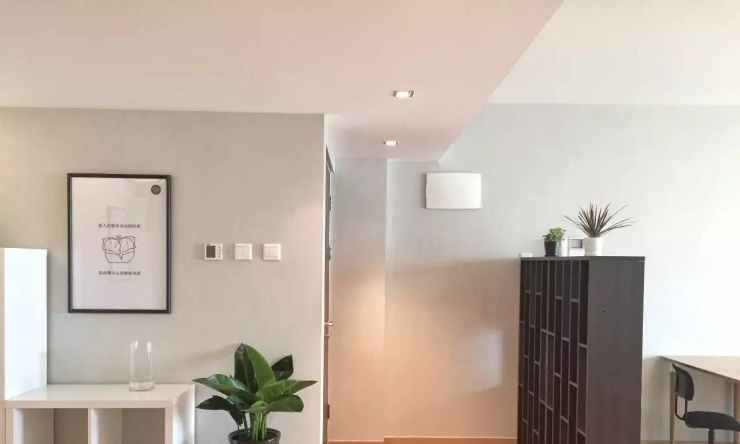好好住APP用户吉祥

吊顶还是因为空间来决定的，人为的原因也有。不是有些空间主人想的自己的空间必须有吊顶才完美，更多的原因是空间来决定的。

首先层高过于高，面积又小的空间，有必要吊顶，这样会使在空间里面的人有足够的安全感，舒适度也会提高。

超大面积的空间也有必要局部吊顶，这样会更好的划分空间的同时又不会因为立墙体遮挡视线。

还有就是特定的空间要有必要的吊顶设计，比如医院手术室，影院等等，还有就是为了达到我们想要的空间效果的时候回做吊顶，比如我们想要的星空顶面。

最后一种是为了用来遮挡结构构件及各种设备管道和装置。

总之，吊顶可以起到空间的层次感，也能很好的划分空间格局，更能增添空间气氛。

圆桌主持人姜波啦

（总结）

吊顶是有自己意义的：

1.功能性上来说，有遮挡顶部设备、管线、梁的作用，也起到安装灯具、中央空调、新风系统的作用；

2.装饰性上来说，有协调你家空间比利的作用，也可以用于塑造空间的风格。

3.不要在层高低于2400mm的情况下还选择吊顶，会略显压抑。不过压抑感因人而异。

所以千万不要为了装吊顶而装吊顶。

客厅、餐厅、主卧、次卧，局部吊顶一共是2100元。卫生间、厨房、生活阳台的集成吊顶（包含灯具和暖风机）一共3800元。

涉及机器，所以使用了轻钢龙骨和石膏板。‌厨房、卫生间就是奥普的包含暖风机套餐，一般的铝扣板吧。集成吊顶感觉隔音效果不太好我家新风感觉有点噪音，在卫生间。好好住APP用户海嘞个绵

吊顶材质一般有：石膏、铝扣板、木、桑拿板、集成吊顶……

我家厕所和厨房做了集成，一个过道做了石膏。我是交给包工头做，就看了总价，不清楚具体多少钱。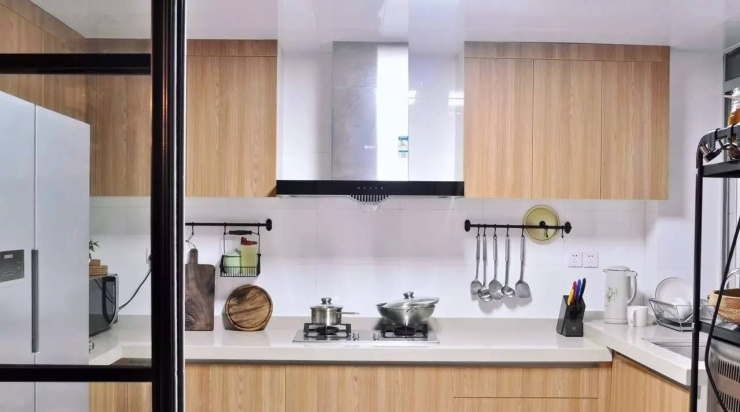我有套房子设计的美岩板吊顶，很像水泥。美岩板按照张算钱，一张大概200来块钱，工人公费另算。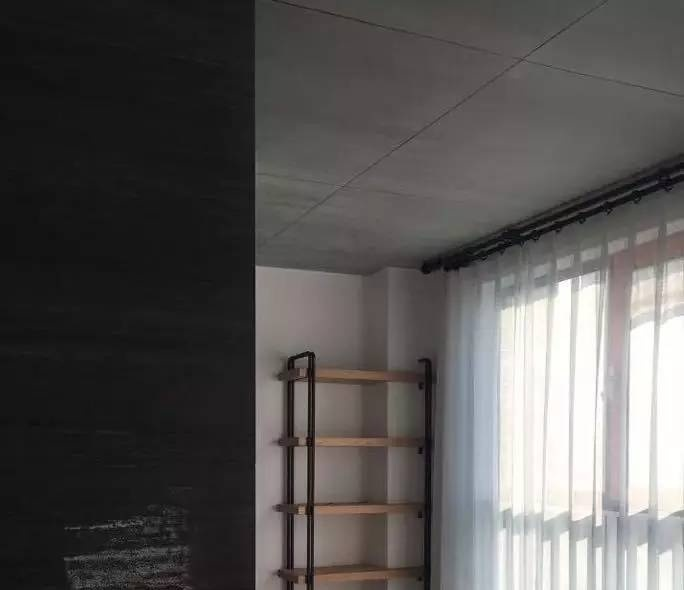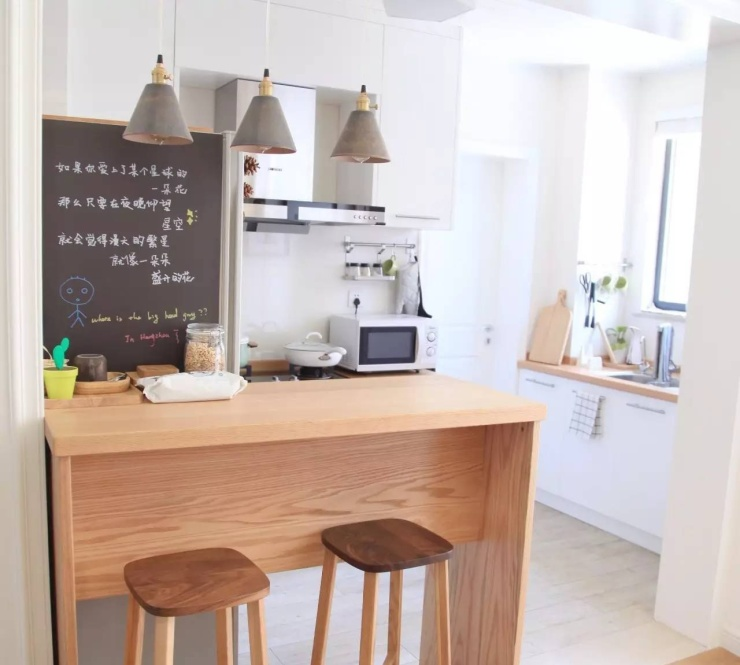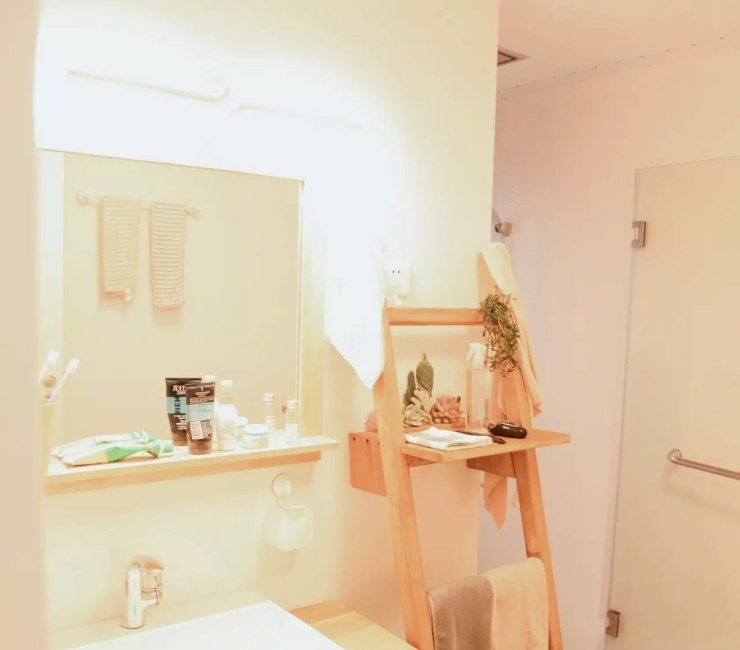常用的手段就是弱化顶部的各种结构，可以用颜色弱化，统一色调。比如说：深灰色，黑色，白色。

还可以反向思维，居然顶部有各种管道，结构暴露，我们可以把这个缺陷视为这个空间的特点和亮点，故意做醒目的色调，或者夸张的颜色搭配，前提是此空间适不适合。

我家没吊顶，挺好，明线直接走，很爽！我家没有吊顶，用挖槽埋线解决那些线的问题。‍‍

‍‍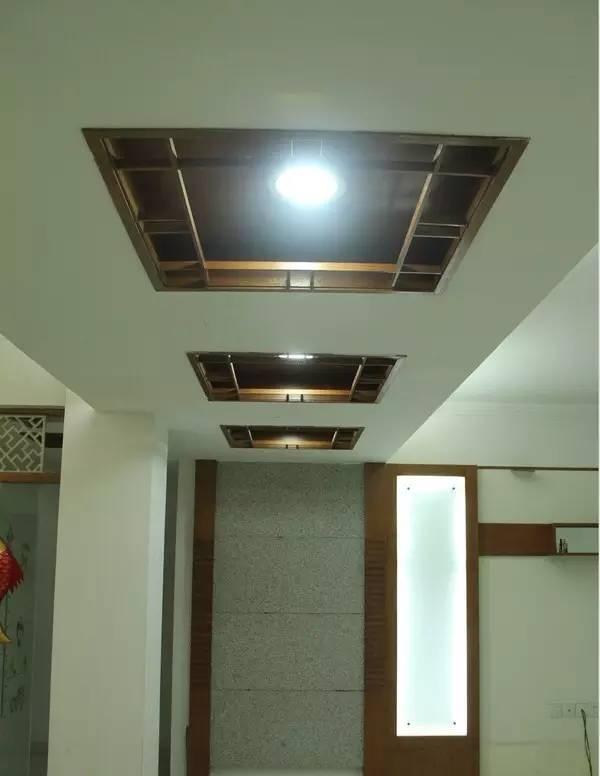‍‍▲施工中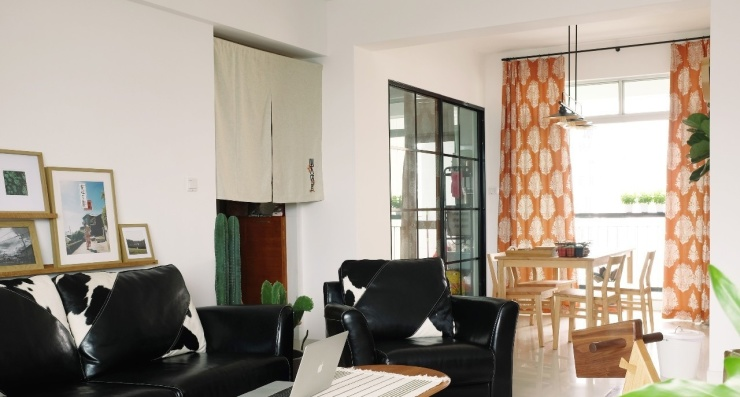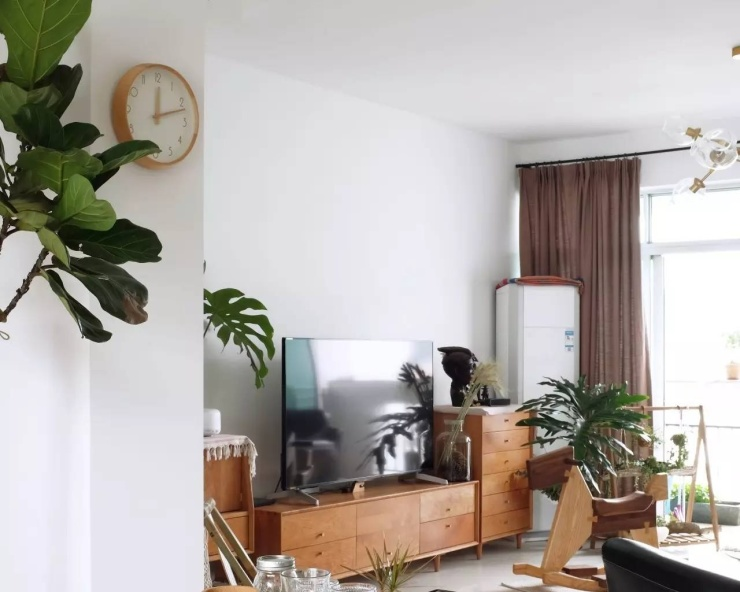其实吊不吊顶主要看个人吧，管道和布线在精心设计下裸露出来也可以很美的哈哈哈。我个人觉得感觉这个吊顶一做，整体风格感觉有点……局限吧…… 感觉定位死了，还是简装修重装饰吧。

应该很少人尝试那种工业风， 管、梁 露出来挺好看的。主要是我原来的业主客厅没有主灯，他的灯主要是电视墙的壁灯，和为了区分客厅和餐厅空间区域做的吊顶，在上面做了灯带，最后我统统敲掉了，从走廊挖了个槽到客厅中间。

刘一汀：现在是比较流行工业风，但是这种风格更适合挑高高的空间。而且施工工艺 管线排布走向都要求比较严格，国内有些施工会达不到，反而难看。

坚决不要听装修公司的忽悠，我们就是血淋淋的教训！

装修公司一定要我们装很复杂的石膏线，因为他们做起来要简单……于是我们回到家，发现吊顶已经装好了，之前还问过我们要什么样的吊顶，我们找了几张图片，都是直角那种，简简单单，事后发现他们一开始就没打算装成我们要的那样的……我们说要把石膏线拆掉，他们说返工很麻烦，要重新拆掉什么的……脸皮薄也就只能忍了。好在整个硬装实在简单，全部白墙，所以石膏线才不算特别突兀。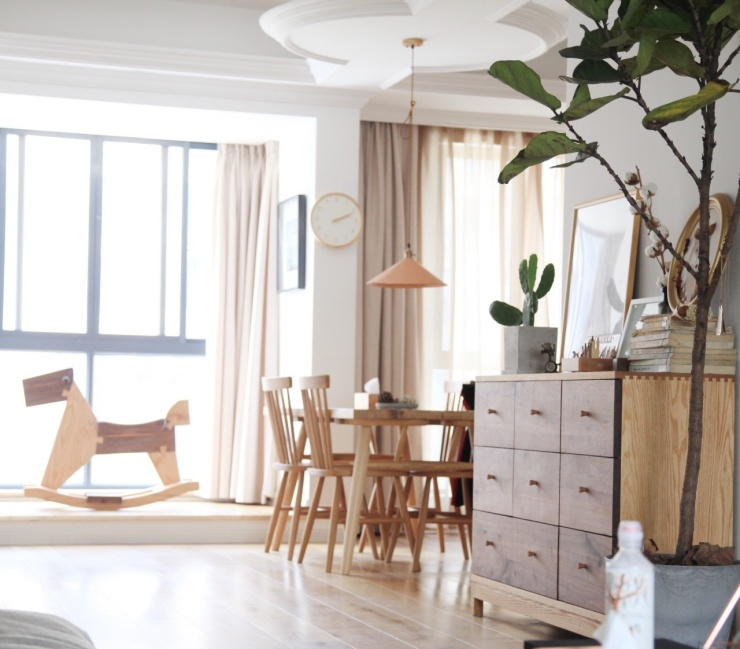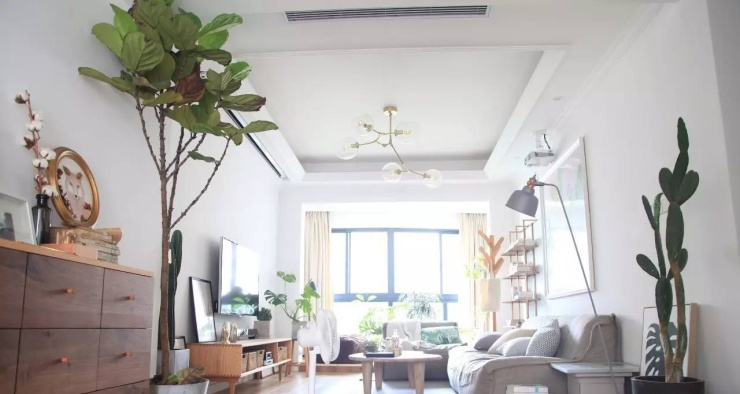`声明：本文由入驻焦点开放平台的作者撰写，除焦点官方账号外，观点仅代表作者本人，不代表焦点立场错误信息举报电话： 400-099-0099，邮箱：jubao@vip.sohu.com，或点此进行意见反馈，或点此进行举报投诉。`A B C D E F G H J K L M N P Q R S T W X Y Z
A - B - C - D - E
• A
• 鞍山
• 安庆
• 安阳
• 安顺
• 安康
• 澳门
• B
• 北京
• 保定
• 包头
• 巴彦淖尔
• 本溪
• 蚌埠
• 亳州
• 滨州
• 北海
• 百色
• 巴中
• 毕节
• 保山
• 宝鸡
• 白银
• 巴州
• C
• 承德
• 沧州
• 长治
• 赤峰
• 朝阳
• 长春
• 常州
• 滁州
• 池州
• 长沙
• 常德
• 郴州
• 潮州
• 崇左
• 重庆
• 成都
• 楚雄
• 昌都
• 慈溪
• 常熟
• D
• 大同
• 大连
• 丹东
• 大庆
• 东营
• 德州
• 东莞
• 德阳
• 达州
• 大理
• 德宏
• 定西
• 儋州
• 东平
• E
• 鄂尔多斯
• 鄂州
• 恩施
F - G - H - I - J
• F
• 抚顺
• 阜新
• 阜阳
• 福州
• 抚州
• 佛山
• 防城港
• G
• 赣州
• 广州
• 桂林
• 贵港
• 广元
• 广安
• 贵阳
• 固原
• H
• 邯郸
• 衡水
• 呼和浩特
• 呼伦贝尔
• 葫芦岛
• 哈尔滨
• 黑河
• 淮安
• 杭州
• 湖州
• 合肥
• 淮南
• 淮北
• 黄山
• 菏泽
• 鹤壁
• 黄石
• 黄冈
• 衡阳
• 怀化
• 惠州
• 河源
• 贺州
• 河池
• 海口
• 红河
• 汉中
• 海东
• 怀来
• I
• J
• 晋中
• 锦州
• 吉林
• 鸡西
• 佳木斯
• 嘉兴
• 金华
• 景德镇
• 九江
• 吉安
• 济南
• 济宁
• 焦作
• 荆门
• 荆州
• 江门
• 揭阳
• 金昌
• 酒泉
• 嘉峪关
K - L - M - N - P
• K
• 开封
• 昆明
• 昆山
• L
• 廊坊
• 临汾
• 辽阳
• 连云港
• 丽水
• 六安
• 龙岩
• 莱芜
• 临沂
• 聊城
• 洛阳
• 漯河
• 娄底
• 柳州
• 来宾
• 泸州
• 乐山
• 六盘水
• 丽江
• 临沧
• 拉萨
• 林芝
• 兰州
• 陇南
• M
• 牡丹江
• 马鞍山
• 茂名
• 梅州
• 绵阳
• 眉山
• N
• 南京
• 南通
• 宁波
• 南平
• 宁德
• 南昌
• 南阳
• 南宁
• 内江
• 南充
• P
• 盘锦
• 莆田
• 平顶山
• 濮阳
• 攀枝花
• 普洱
• 平凉
Q - R - S - T - W
• Q
• 秦皇岛
• 齐齐哈尔
• 衢州
• 泉州
• 青岛
• 清远
• 钦州
• 黔南
• 曲靖
• 庆阳
• R
• 日照
• 日喀则
• S
• 石家庄
• 沈阳
• 双鸭山
• 绥化
• 上海
• 苏州
• 宿迁
• 绍兴
• 宿州
• 三明
• 上饶
• 三门峡
• 商丘
• 十堰
• 随州
• 邵阳
• 韶关
• 深圳
• 汕头
• 汕尾
• 三亚
• 三沙
• 遂宁
• 山南
• 商洛
• 石嘴山
• T
• 天津
• 唐山
• 太原
• 通辽
• 铁岭
• 泰州
• 台州
• 铜陵
• 泰安
• 铜仁
• 铜川
• 天水
• 天门
• W
• 乌海
• 乌兰察布
• 无锡
• 温州
• 芜湖
• 潍坊
• 威海
• 武汉
• 梧州
• 渭南
• 武威
• 吴忠
• 乌鲁木齐
X - Y - Z
• X
• 邢台
• 徐州
• 宣城
• 厦门
• 新乡
• 许昌
• 信阳
• 襄阳
• 孝感
• 咸宁
• 湘潭
• 湘西
• 西双版纳
• 西安
• 咸阳
• 西宁
• 仙桃
• 西昌
• Y
• 运城
• 营口
• 盐城
• 扬州
• 鹰潭
• 宜春
• 烟台
• 宜昌
• 岳阳
• 益阳
• 永州
• 阳江
• 云浮
• 玉林
• 宜宾
• 雅安
• 玉溪
• 延安
• 榆林
• 银川
• Z
• 张家口
• 镇江
• 舟山
• 漳州
• 淄博
• 枣庄
• 郑州
• 周口
• 驻马店
• 株洲
• 张家界
• 珠海
• 湛江
• 肇庆
• 中山
• 自贡
• 资阳
• 遵义
• 昭通
• 张掖
• 中卫

1室1厅1厨1卫1阳台

1
2
3
4
5

0
1
2

1

1

0
1
2
3报名成功，资料已提交审核A B C D E F G H J K L M N P Q R S T W X Y Z
A - B - C - D - E
• A
• 鞍山
• 安庆
• 安阳
• 安顺
• 安康
• 澳门
• B
• 北京
• 保定
• 包头
• 巴彦淖尔
• 本溪
• 蚌埠
• 亳州
• 滨州
• 北海
• 百色
• 巴中
• 毕节
• 保山
• 宝鸡
• 白银
• 巴州
• C
• 承德
• 沧州
• 长治
• 赤峰
• 朝阳
• 长春
• 常州
• 滁州
• 池州
• 长沙
• 常德
• 郴州
• 潮州
• 崇左
• 重庆
• 成都
• 楚雄
• 昌都
• 慈溪
• 常熟
• D
• 大同
• 大连
• 丹东
• 大庆
• 东营
• 德州
• 东莞
• 德阳
• 达州
• 大理
• 德宏
• 定西
• 儋州
• 东平
• E
• 鄂尔多斯
• 鄂州
• 恩施
F - G - H - I - J
• F
• 抚顺
• 阜新
• 阜阳
• 福州
• 抚州
• 佛山
• 防城港
• G
• 赣州
• 广州
• 桂林
• 贵港
• 广元
• 广安
• 贵阳
• 固原
• H
• 邯郸
• 衡水
• 呼和浩特
• 呼伦贝尔
• 葫芦岛
• 哈尔滨
• 黑河
• 淮安
• 杭州
• 湖州
• 合肥
• 淮南
• 淮北
• 黄山
• 菏泽
• 鹤壁
• 黄石
• 黄冈
• 衡阳
• 怀化
• 惠州
• 河源
• 贺州
• 河池
• 海口
• 红河
• 汉中
• 海东
• 怀来
• I
• J
• 晋中
• 锦州
• 吉林
• 鸡西
• 佳木斯
• 嘉兴
• 金华
• 景德镇
• 九江
• 吉安
• 济南
• 济宁
• 焦作
• 荆门
• 荆州
• 江门
• 揭阳
• 金昌
• 酒泉
• 嘉峪关
K - L - M - N - P
• K
• 开封
• 昆明
• 昆山
• L
• 廊坊
• 临汾
• 辽阳
• 连云港
• 丽水
• 六安
• 龙岩
• 莱芜
• 临沂
• 聊城
• 洛阳
• 漯河
• 娄底
• 柳州
• 来宾
• 泸州
• 乐山
• 六盘水
• 丽江
• 临沧
• 拉萨
• 林芝
• 兰州
• 陇南
• M
• 牡丹江
• 马鞍山
• 茂名
• 梅州
• 绵阳
• 眉山
• N
• 南京
• 南通
• 宁波
• 南平
• 宁德
• 南昌
• 南阳
• 南宁
• 内江
• 南充
• P
• 盘锦
• 莆田
• 平顶山
• 濮阳
• 攀枝花
• 普洱
• 平凉
Q - R - S - T - W
• Q
• 秦皇岛
• 齐齐哈尔
• 衢州
• 泉州
• 青岛
• 清远
• 钦州
• 黔南
• 曲靖
• 庆阳
• R
• 日照
• 日喀则
• S
• 石家庄
• 沈阳
• 双鸭山
• 绥化
• 上海
• 苏州
• 宿迁
• 绍兴
• 宿州
• 三明
• 上饶
• 三门峡
• 商丘
• 十堰
• 随州
• 邵阳
• 韶关
• 深圳
• 汕头
• 汕尾
• 三亚
• 三沙
• 遂宁
• 山南
• 商洛
• 石嘴山
• T
• 天津
• 唐山
• 太原
• 通辽
• 铁岭
• 泰州
• 台州
• 铜陵
• 泰安
• 铜仁
• 铜川
• 天水
• 天门
• W
• 乌海
• 乌兰察布
• 无锡
• 温州
• 芜湖
• 潍坊
• 威海
• 武汉
• 梧州
• 渭南
• 武威
• 吴忠
• 乌鲁木齐
X - Y - Z
• X
• 邢台
• 徐州
• 宣城
• 厦门
• 新乡
• 许昌
• 信阳
• 襄阳
• 孝感
• 咸宁
• 湘潭
• 湘西
• 西双版纳
• 西安
• 咸阳
• 西宁
• 仙桃
• 西昌
• Y
• 运城
• 营口
• 盐城
• 扬州
• 鹰潭
• 宜春
• 烟台
• 宜昌
• 岳阳
• 益阳
• 永州
• 阳江
• 云浮
• 玉林
• 宜宾
• 雅安
• 玉溪
• 延安
• 榆林
• 银川
• Z
• 张家口
• 镇江
• 舟山
• 漳州
• 淄博
• 枣庄
• 郑州
• 周口
• 驻马店
• 株洲
• 张家界
• 珠海
• 湛江
• 肇庆
• 中山
• 自贡
• 资阳
• 遵义
• 昭通
• 张掖
• 中卫• 手机• 分享
• 设计
免费设计
• 计算器
装修计算器
• 入驻
合作入驻
• 联系
联系我们
• 置顶
返回顶部